# Search

About 22 Search Results Matching Types of Worksheet, Worksheet Section, Generator, Generator Section, Subjects matching Geometry, Similar to Math Worksheets for Kids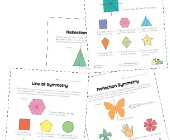## Symmetry Worksheets

Help kids learn about the concept of symmetry with...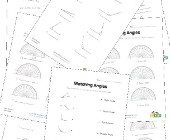## Angles Worksheets

Help kids learn about angles with this collection ...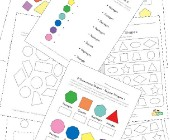## Geometry Worksheets

Help kids learn the early basics of geometry inclu...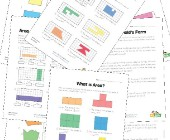## Area and Perimeter Worksheets

Help kids learn to calculate the area and perimete...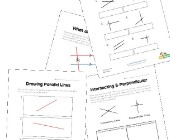## Parallel, Perpendicular and Intersecting Lines Worksheets

Help kids learn about lines including parallel lin...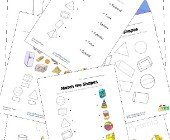## 3 Dimensional Shapes Worksheets

Check out our collection of 3D shapes worksheets f...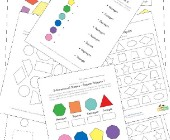## 2 Dimensional Shapes Worksheets

Check out our collection of 2D shapes worksheets f...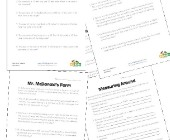## Area and Perimeter Word Problems

This collection of area and perimeter worksheets c...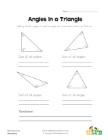## Angles in a Triangle Worksheet

Kids learn that the angles of a triangle always ad...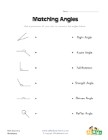## Matching Angles Worksheet

Draw a line to match each of the angles with the t...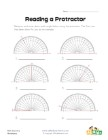## Reading a Protractor Worksheet 2

Read each protractor and write down the angles the...